# RD Sharma Solutions (Ex.1.2): Rational Numbers Notes | Study RD Sharma Solutions for Class 8 Mathematics - Class 8

## Class 8: RD Sharma Solutions (Ex.1.2): Rational Numbers Notes | Study RD Sharma Solutions for Class 8 Mathematics - Class 8

The document RD Sharma Solutions (Ex.1.2): Rational Numbers Notes | Study RD Sharma Solutions for Class 8 Mathematics - Class 8 is a part of the Class 8 Course RD Sharma Solutions for Class 8 Mathematics.
All you need of Class 8 at this link: Class 8

Question 1: Verify commutativity of addition of rational numbers for each of the following pairs of rational numbers:

(i) -11/5 and 4/7

(ii) 4/9 and 7/-1249 and 7-12

(iii)  -3/5 and -2/-15

(iv) 2/-7 and 12/-35

(v) 4 and -3/5

(vi) -4 and 4/-7

Answer :Commutativity of the addition of rational numbers means that if a/b and c/d are two rational numbers, then a / b + c / d = / d + a / b .(i)

We have -11/5 and 4/7.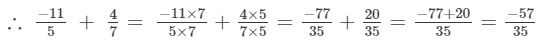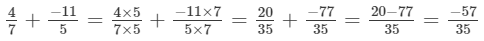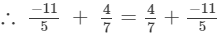Hence verified.(ii)

We have 4/9 and 7/-12.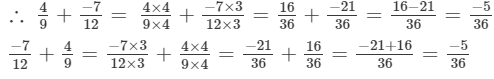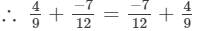Hence verified. (iii)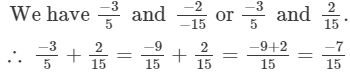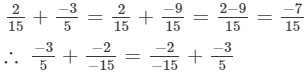Hence verified. (iv)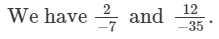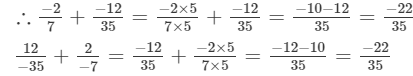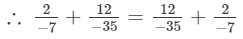Hence verified. (v)

We have 4 and -3/5.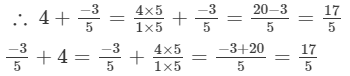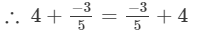Hence verified. (vi)

We have -4 and 4/-7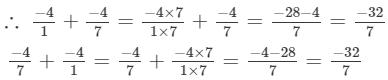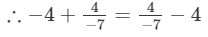Hence verified.

Question 2: Verify associativity of addition of rational numbers i.e., (x + y) + z = x + (y + z), when: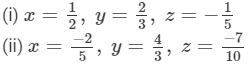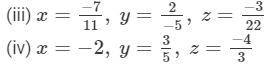Answer : We have to verify that (x+y)+z = x+(y+z).

(i)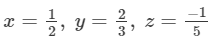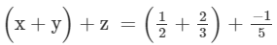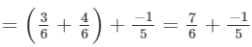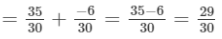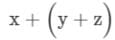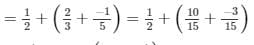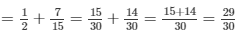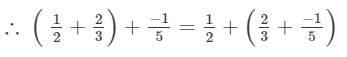Hence verified. (ii)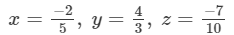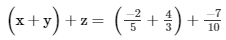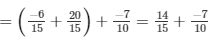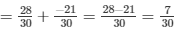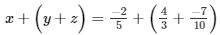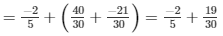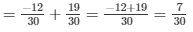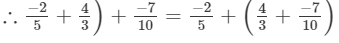Hence verified. (iii)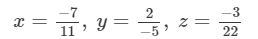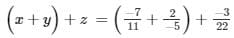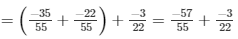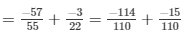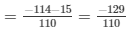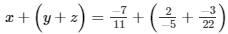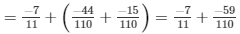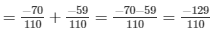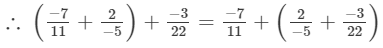Hence verified. (iv)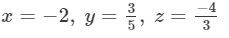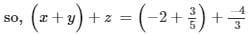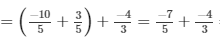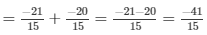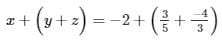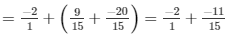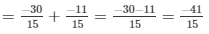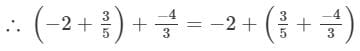Hence verified.

Question 3: Write the additive inverse of each of the following rational numbers: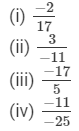(i)Additive inverse is the negative of the given number.So, additive inverse of −2/17 is 2/17.

(ii) Additive inverse is the negative of the given number.So, additive inverse of 3/-11 is 3/11.

(iii) Additive inverse is the negative of the given number.So, additive inverse of -17/5 is 17/5.

(iv) Additive inverse is the negative of the given number.So, additive inverse of  -11/-25 is -11/25.

Question 4: Write the negative (additive inverse) of each of the following:

(i) -2/5-25
(ii) 7/-97-9
(iii) -16/13
(iv) -5/1
(v) 0
(vi) 1
(vii) −1

Answer : (i) Negative of −2/5 is 2/5.

(ii) Negative of 7/−9 is 7/9.

(iii) Negative of −16/13 is 16/13.

(iv) Negative of −5/1 is 5/1.

(v) Negative value of 0 is 0.

(vi) Negative of 1 is −1.

(vi) Negative of −1 is 1.

Question 5: Using commutativity and associativity of addition of rational numbers, express each of the following as a rational number: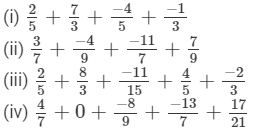(i) We have: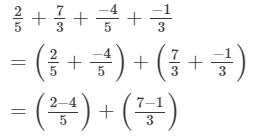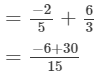= 24/15= 8/5

(ii) We have: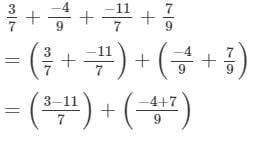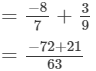= -51/63= -17/21

(iii) We have: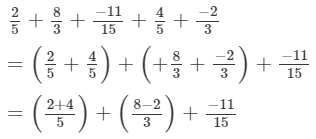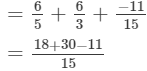= 37/15

(iv) We have: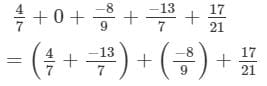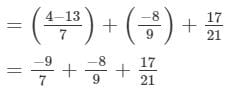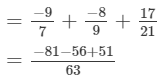= -86/63

Question 6: Re-arrange suitably and find the sum in each of the following: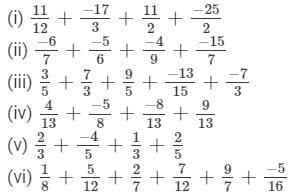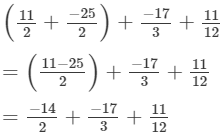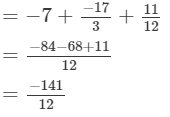= -47/4

(ii)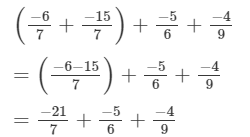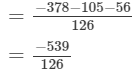= -77/18

(iii)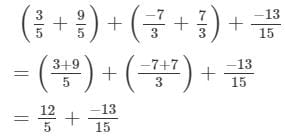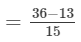= 23/15

(iv)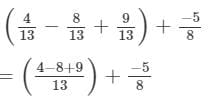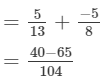= -25/104

(v)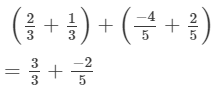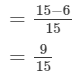= 3/5

(vi)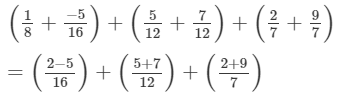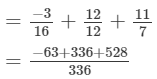= 801/336

= 267/112

The document RD Sharma Solutions (Ex.1.2): Rational Numbers Notes | Study RD Sharma Solutions for Class 8 Mathematics - Class 8 is a part of the Class 8 Course RD Sharma Solutions for Class 8 Mathematics.
All you need of Class 8 at this link: Class 8Use Code STAYHOME200 and get INR 200 additional OFF

## RD Sharma Solutions for Class 8 Mathematics

88 docs

Track your progress, build streaks, highlight & save important lessons and more!

,

,

,

,

,

,

,

,

,

,

,

,

,

,

,

,

,

,

,

,

,

;